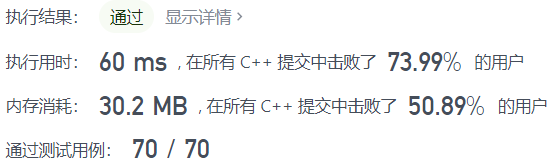# leedcode 128. 最长连续序列（哈希）

## 示例

0 <= nums.length <= 105
-109 <= nums[i] <= 109

## 题解

• 哈希 map
• 看到需要 o(n) 的复杂度，以为只是遍历一遍，没有思路。想了五六分钟，想到就算遍历两遍，也是 o(n) 的复杂度，思路代码都想出来了。
• 比如  nums = [100,4,200,1,3,2]  第一遍遍历，mp=1，mp=1，mp=1，mp=1，mp=1，mp=1。第二次遍历，mp=1，看 100 以前和以后还有没有连续的数，mp=1，把 mp标记为2。mp==0 不存在，mp==0 不存在 ，所以此时最大连续为 1。遍历到 4 时，看 4以前和以后还有没有连续的数，mp==0 不存在，再看 4 以前的数，mp=1，mp=1，mp=1，并把它们标记为 2，mp=2，mp=2，mp=2，mp=2，所以此时最大连续为 4。200 同理。遍历到 1 时，由于 mp=2，说明已经遍历过第二遍了，不用再遍历了。后面的也同理。所以，每个数只被遍历 2 此，时间复杂度为 o(2n)，即为  o(n)。
class Solution {
public:
int longestConsecutive(vector<int>& nums) {
unordered_map<int,int>mp;
int ans=0;
int n = nums.size();
for(int i=0;i<n;i++){//nums中有的数都标记为 1
mp[nums[i]]=1;
}
for(int i=0;i<n;i++){//再遍历一遍nums
if(mp[nums[i]]==1){
int count=0;
for(int j=nums[i];j<1000000005;j++){//往右最大到多少
if(mp[j]==1){//如果第二遍没遍历过且存在
mp[j]=2;//标记第二遍已经遍历过
count++;
}else{
break;
}
}
for(int j=nums[i]-1;j>-1000000005;j--){//往左最小到多少
if(mp[j]==1){//如果第二遍没遍历过且存在
mp[j]=2;//标记第二遍已经遍历过
count++;
}else{
break;
}
}
ans=max(ans,count);
}
}
return ans;
}
};• 官方也是哈希，用的是 set，代码更简洁
• 第一次遍历用 set 标记，再去重，第二次遍历，每次只检查这个数是否是连续序列的第一个数，也就是这个数 x 在 set 中且它的前驱数 x-1不在 set 中。如果这个数是连续序列的第一个数，那么向后遍历有多少个数在数组中，即是否有一个 x, x+1, x+2,……, x+y 的连续序列，其长度为 y+1。
class Solution {
public:
int longestConsecutive(vector<int>& nums) {
unordered_set<int> num_set;
for (const int& num : nums) {
num_set.insert(num);//放入 set 中
}

int longestStreak = 0;

for (const int& num : num_set) {
if (!num_set.count(num - 1)) {//检查这个数是否是连续序列的第一个数
int currentNum = num;
int currentStreak = 1;//此时长度

while (num_set.count(currentNum + 1)) {//向后遍历有多少个连续数
currentNum += 1;
currentStreak += 1;//长度+1
}

longestStreak = max(longestStreak, currentStreak);
}
}

return longestStreak;
}
};

posted on 2021-09-20 15:10  蔡军帅  阅读(33)  评论(0编辑  收藏  举报